# Fractal facts for kids

Kids Encyclopedia Facts
(Redirected from Fractal geometry)

A fractal is any pattern, that when seen as an image, produces a picture, which when zoomed into will still make the same picture. It can be cut into parts which look like a smaller version of the picture that was started with. The word fractal was made by Benoît Mandelbrot in 1975 from the Latin word fractus, which means "broken" or "fractured". A simple example is a tree that branches into smaller branches, and those branches into smaller branches and so on. Fractals are not only beautiful, but also have many practical applications.

## Examples

There are many types of fractals, made in a large variety of ways. One example is the Sierpinski triangle, where there are an infinite number of small triangles inside the large one. Another example is the Mandelbrot set, named for Benoît Mandelbrot. The Sierpinksi triangle is constructed using patterns, but the Mandelbrot set is based on an equation.

There are also many natural examples of fractals in nature including trees, snowflakes, some vegetables and coastlines.

### The Koch Curve

The Koch Curve is a simple example of a fractal. First, start with part of a straight line - called a straight line segment. Cut the line into 3 same-sized pieces. Get rid of the middle of those pieces, and put in the top part of a triangle with sides which are the same length as the bit to cut out. We now have 4 line segments which are touching at the ends. We can now do what we just did to the first segment to each of the 4 bits. We can now do the same thing again and again to all the bits we end up with. We now do this forever and look at what we end up with.

The length of the Koch Curve is infinity, and the area of the Koch Curve is zero. This is quite strange. A line segment (with dimension 1) could have a length of 1, but it has an area of 0. A square of length 1 and width 1 (with dimension 2) will have area 1 and length of infinity.

### Similarity dimension

So, the Koch Curve seems to be bigger than something of dimension 1, and smaller than something of dimension 2. The idea of the similarity dimension is to give a dimension which gives a better idea of length or area for fractals. So, for a Koch Curve, we want a dimension between 1 and 2.

The Koch Curve can be cut into four pieces, each of which are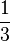$\frac{1}{3}$ of the size of the original. We call the number of pieces that a fractal can be cut into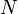$N$, and we call the size difference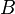$B$. We put those into the equation: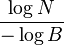$\frac{\log N}{-\log B}$

Where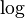$\log$ is the logarithm of a number. This number is the Hausdorff Dimension of the fractal. In the Koch Curve, this is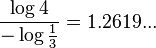$\frac{\log 4}{-\log \frac{1}{3}}=1.2619...$ as we wanted.

The Koch Curve is one of the simplest fractal shapes, and so its dimension is easy to work out. Its similarity dimension and Hausdorff dimension are both the same. This is not true for more complex fractals.

### Koch snowflake

The Koch snowflake (or Koch star) is the same as the Koch curve, except it starts with an equilateral triangle instead of a line segment.

## Uses

Fractals have many applications e.g. in biology (lung, kidneys, heart rate variability, etc...), in earthquakes, in finance where it is related to the so called heavy tail distributions and in physics. This indicates that fractals should be studied to understand why fractals are so frequent in nature.

Some fractals exist only for artistic reasons, but others are very useful. Fractals are very efficient shapes for radio antennas and are used in computer chips to efficiently connect all the components. Also, coastlines can be thought of as fractals.

## Images for kidsFractal Facts for Kids. Kiddle Encyclopedia.